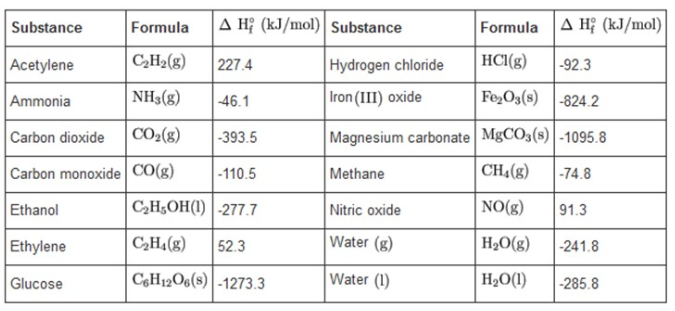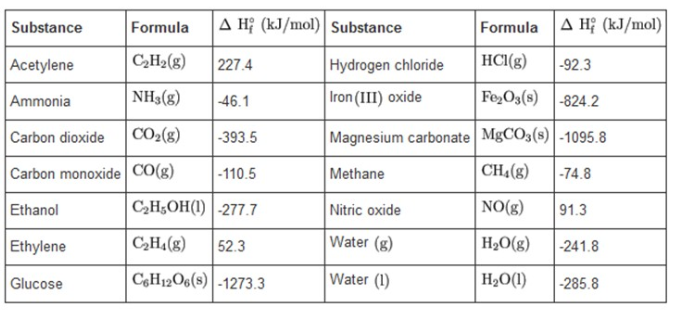# Problem: Use the information in the table to calculate ΔH° in kilojoules for the photosynthesis of glucose (C6H12O6) and O2 from CO2 and liquid H2O, a reaction carried out by all green plants. TABLE Standard Heats of Formation for Some Common Substances at 25°C Express your answer using four significant figures.

###### FREE Expert Solution

We need to find the ΔH° or (ΔH°rxn) in kJ for the photosynthesis reaction of glucose (C6H12O6) and O2 from CO2 and liquid H2O, a reaction carried out by all green plants.

Recall that the equation for ΔH°rxn is:

$\overline{){\mathbf{∆}}{\mathbit{H}}{{\mathbf{°}}}_{{\mathbf{rxn}}}{\mathbf{=}}{\mathbf{\left(}}{\mathbit{p}}{\mathbit{r}}{\mathbit{o}}{\mathbit{d}}{\mathbit{u}}{\mathbit{c}}{\mathbit{t}}{\mathbit{s}}{\mathbf{\right)}}{\mathbf{-}}{\mathbf{\left(}}{\mathbit{r}}{\mathbit{e}}{\mathbit{a}}{\mathbit{c}}{\mathbit{t}}{\mathbit{a}}{\mathbit{n}}{\mathbit{t}}{\mathbit{s}}{\mathbf{\right)}}}$
We will use the following table for the standard heats of formation, ΔH°f:

TABLE Standard Heats of Formation for Some Common Substances at 25°C:84% (226 ratings)###### Problem Details

Use the information in the table to calculate ΔH° in kilojoules for the photosynthesis of glucose (C6H12O6) and O2 from CO2 and liquid H2O, a reaction carried out by all green plants.

TABLE Standard Heats of Formation for Some Common Substances at 25°C